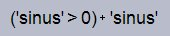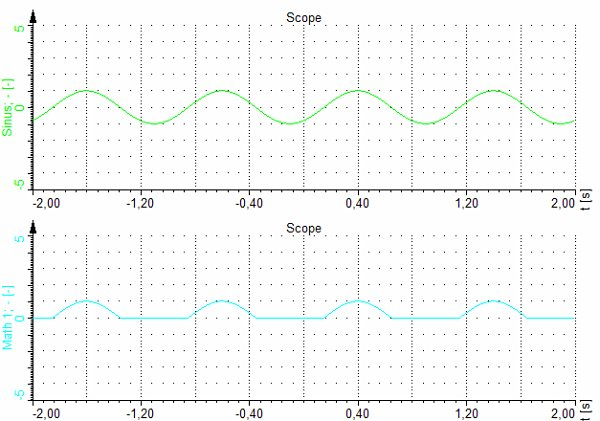# Example of logic functions

The result of logic operators is always 0 or 1. Therefore we can use this in further calculations:The first part of the following equation will return 1 when the input channel ‘sinus’ is positive and the value of 0 when the sinus will be negative. Multiplying this result with the original signal will result in cutting the negative part of the signal.